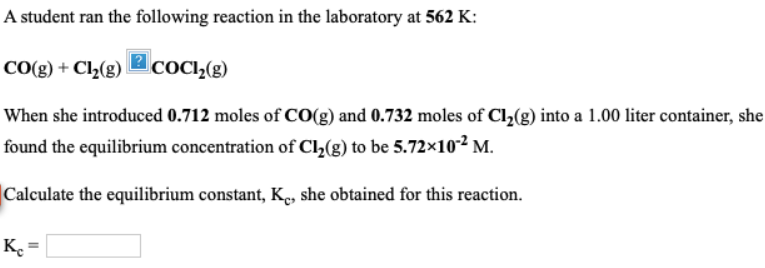# A student ran the following reaction in the laboratory at 562 K: CO(g) + Cl2(g) -> COCl2(g) When she introduced 0.712 moles of CO(g) and 0.732 moles of Cl2(g) into a 1.00 liter container, she found the equilibrium concentration of Cl2(g) to be 5.72 x 10^-2 M. Calculate the equilibrium constant, Kc, she obtained for this reaction. Kc = ___